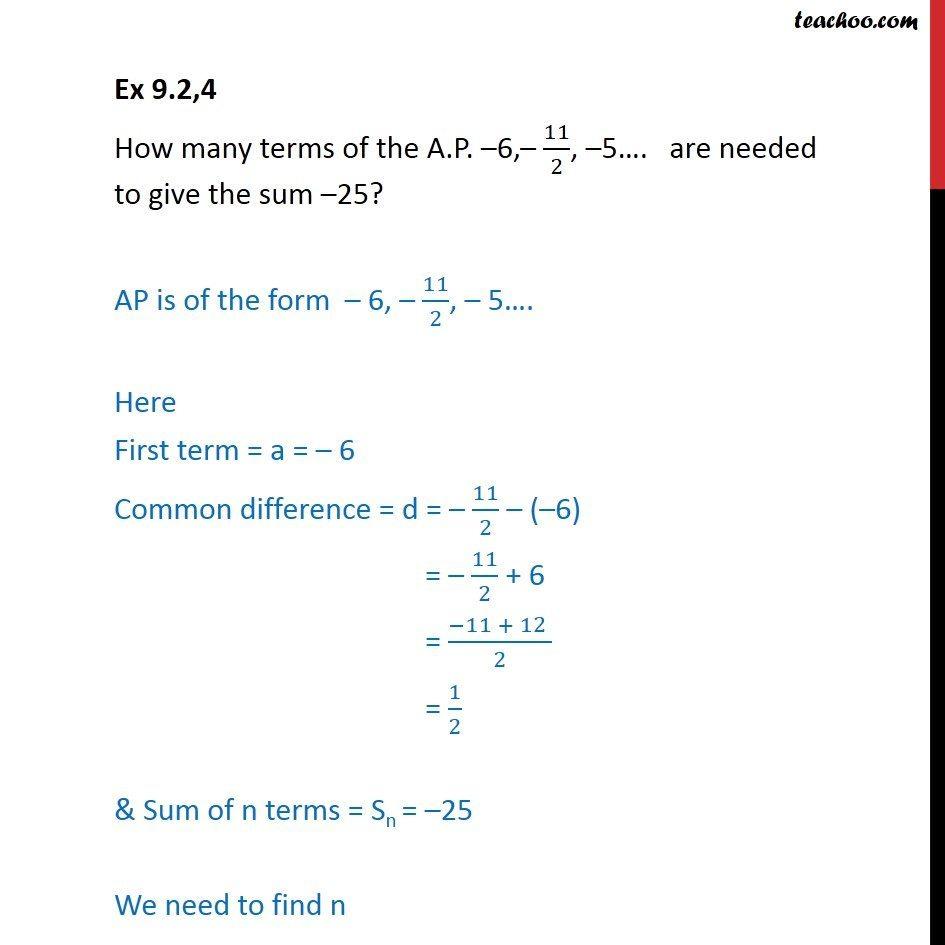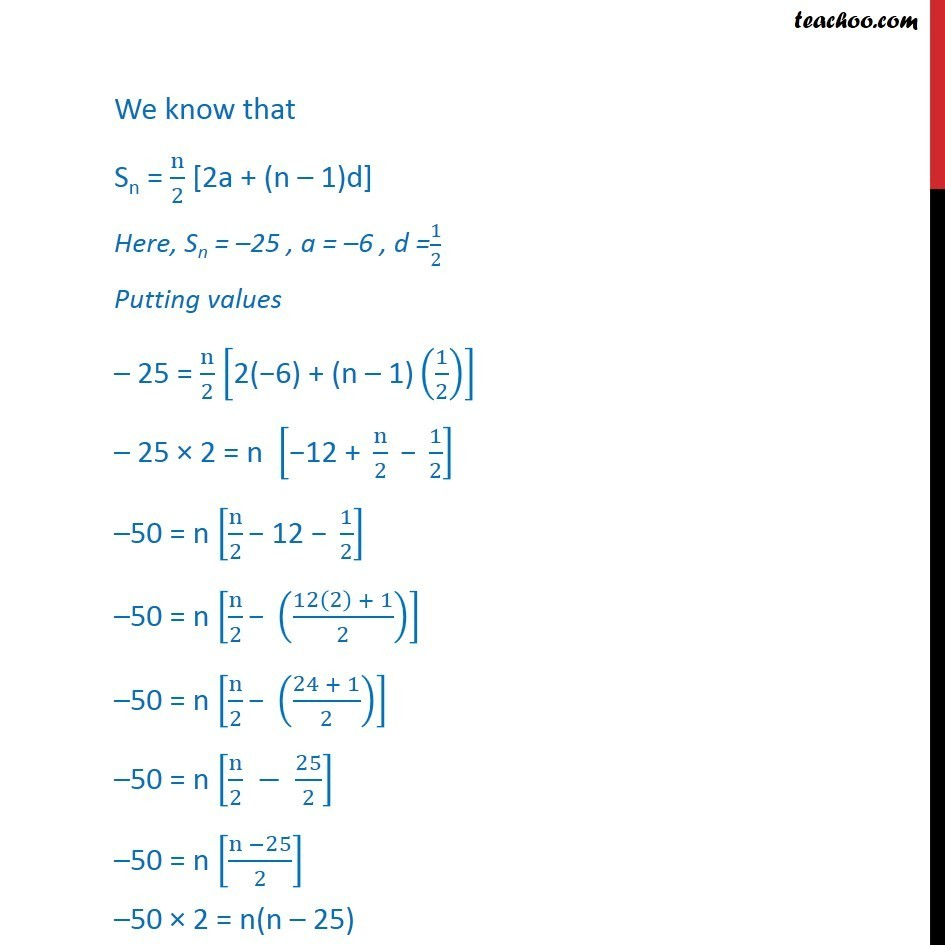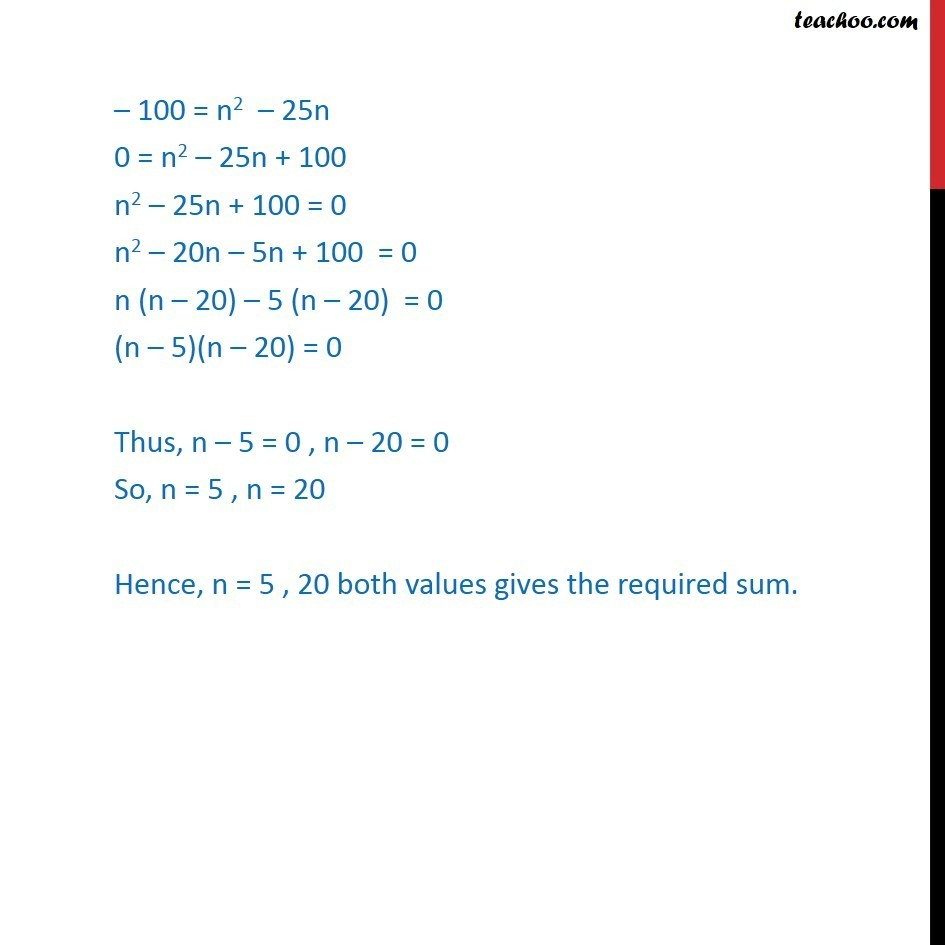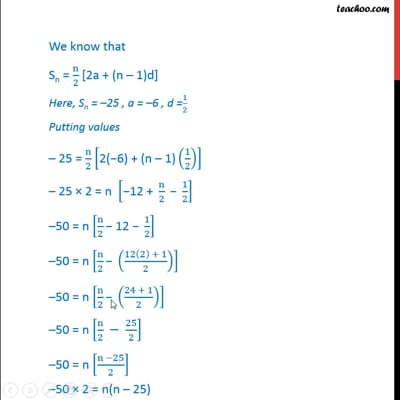Ex 9.2

Chapter 9 Class 11 Sequences and Series
Serial order wiseThis video is only available for Teachoo black users

Solve all your doubts with Teachoo Black (new monthly pack available now!)

### Transcript

Ex 9.2,4 How many terms of the A.P. 6, 11/2, 5 . are needed to give the sum 25? AP is of the form 6, 11/2, 5 . Here First term = a = 6 Common difference = d = 11/2 ( 6) = 11/2 + 6 = ( 11 + 12 )/2 = 1/2 & Sum of n terms = Sn = 25 We need to find n We know that Sn = n/2 [2a + (n 1)d] Here, Sn = 25 , a = 6 , d =1/2 Putting values 25 = n/2 ["2( 6) + (n 1)" (1/2)] 25 2 = n [" 12 + " n/2 " " 1/2] 50 = n [n/2 " 12 " 1/2] 50 = n [n/2 " " ((12(2) + 1)/2)] 50 = n [n/2 " " ((24 + 1)/2)] 50 = n [n/2 25/2] 50 = n [(n 25)/2] 50 2 = n(n 25) 100 = n2 25n 0 = n2 25n + 100 n2 25n + 100 = 0 n2 20n 5n + 100 = 0 n (n 20) 5 (n 20) = 0 (n 5)(n 20) = 0 Thus, n 5 = 0 , n 20 = 0 So, n = 5 , n = 20 Hence, n = 5 , 20 both values gives the required sum.